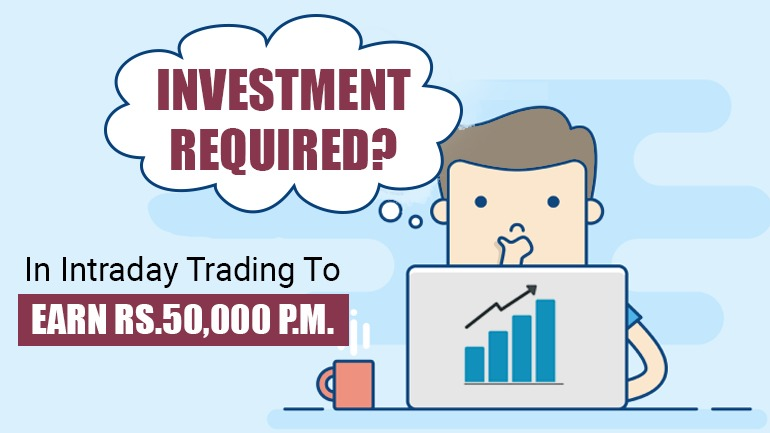We writing this blog as this is the frequently asked logical question.

Let’s see How Many would answer this question.

1. Speculator Mindset and over-confident traders would say: We require approx. just 20% of our trade size, remaining would be funded by broker as we receive margins.
2. If someone is conservative and thinks about risk then he would say, we just need the Max. Risk amount we can bear. I am able to bear this much risk so it is my capital size, these traders are one step better than speculators as at least they think about Risk Mgt. but can it achieve your targeted Profit?

Now to get actual answer of Capital Required, Let’s first understand first what is Capital in Stock Market.

Capital required is Total Risk we need to take to achieve desired Profits. Capital Required is not Risk we wish to take, its Risk we need to take.

Capital Required is not Trade Size, we may sometimes leverage in our intraday positions. but how much leverage we can take?

Leverage Allowed= Our Total Trade Size – Capital Required

So how to calculate Capital Required? Actually, how much capital we require is not dependent on nature of trader aggressive or conservative.

It depends on the 3 factors:

1. Profit Required
2. Statistics of Our Trading Strategy (Actual OR Back tested for minimum 100 Trades) &
3. Our Risk Profile

Let’s elaborate with simple example:

1st Factor: Profit Required

Here we assume we require Profit of Rs. 50,000/-p.m. so 6 Lacs p.a.

Assuming fix and variable Exps. = Rs. 1 Lac p.a.

So gross Profit: Rs. 7 Lacs p.a.

2nd Factor: Statistics of Trading Strategy

1. No of Possible trades in a year = Approx. 528 (2 trades a day * Approx. 22 days *12 months)
2. Reward: Risk = 2
3. Win Rate = 60%

Now we have to calculate Required Expectancy Rate i.e. Average Profit Required Per Trade (Total Profit/ Total Trades) which comes Rs. 1,326 (7 lacs/528).

So, now we have three figures with us Average Profit Required Per Trade, Win Rate and Reward: Risk. Using these data and with the help of Expectancy Formula, we can Find Avg. profit and Avg. loss per trade we have to set while actual trading.

We have calculated above two figures using formula of expectancy:

= (Probability of Win * Average Win) – (Probability of Loss * Average Loss)

3rd Factor: Our Risk Profile

As we have come up with the Risk per trade, now we have to find total Risk which is also known as Capital Required.  So, what should be Total Risk? We have a simple Trading Rule we cannot risk More than 1% of our capital in one day (Total of all trades in one day). That is, we should be in the game even if we incur loss for 100 consecutive days.

So according to above statistics if we risk 1,657 per trade to achieve profits on our trading strategy then we must have capital of Rs 1,65,720/-

Only this much capital required to Make 7 Lacs p.a.?

This is all paper Work yes

Why?

Practical figures when we trade comes very different then paper work we did.

Let’s understand Why Actual figures are many times differ from Planned figures.

1st reason:

You say you will make 528 trades in a year but actually you may just take 400 trades yearly. May be due to market consolidation or may be due to weak psychology you fail to trade many days.

2nd Reason:

Now out of these 400 trades, your 200 trades may not hit SL or Target and just closes near Cost or maybe you have to exit those trades later. So now you are just left with 200 trades with actual Profit or loss.

More Above all, it’s very tough and requires lots of practice to make accuracy of 60% with Reward: Risk of 2 times. Very few of traders are able to get such figures in reality.

Many of our clients use to ask us do u have 80% accuracy, 90% accuracy, all is bullshit. Even with 60% accuracy and 2:1 Reward: Risk can do miracles if followed sincerely with good number of trades, consistently. It could give wonderful ROI.